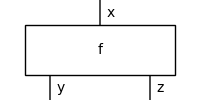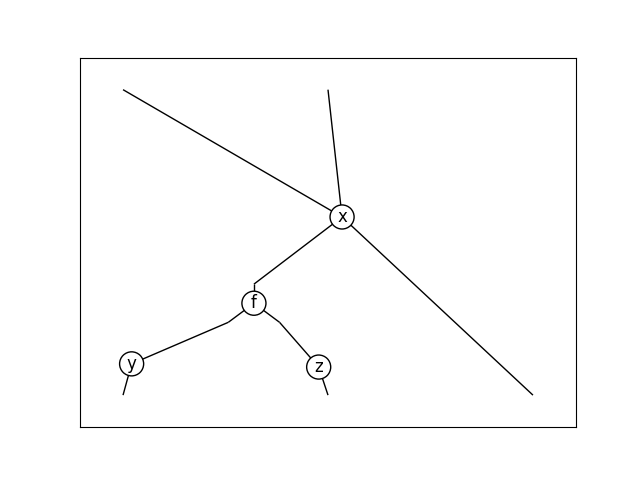# Diagram#

class discopy.hypergraph.Diagram(dom, cod, boxes, wires, spider_types=None)[source]#

Diagram in a hypergraph category.

Parameters
• dom (frobenius.Ty) – The domain of the diagram, i.e. its input.

• cod (frobenius.Ty) – The codomain of the diagram, i.e. its output.

• boxes (tuple[Box, ...]) – The boxes inside the diagram.

• wires (tuple[Any]) – List of wires from ports to spiders.

• spider_types (Mapping[Any, Ty]) – Mapping[Any, frobenius.Ty] Mapping from spiders to atomic types, if `None` then this is computed from the types of ports.

Note

The wires go from ports to spiders, they are given as a list of length:

```len(dom) + sum(len(box.dom) + len(box.cod) for box in boxes) + len(cod)
```

The values themselves don’t matter, they are simply labels for the spiders. We must have `len(types) >= len(set(wires))`, the spiders that don’t appear as the target of a wire are scalar spiders, i.e. with zero legs.

Abstractly, a hypergraph diagram can be seen as a cospan for the boundary:

```range(len(dom)) -> range(n_spiders) <- range(len(cod))
```

together with a cospan for each box in boxes:

```range(len(box.dom)) -> range(n_spiders) <- range(len(box.cod))
```

Composition of two hypergraph diagram is given by the pushout of the span:

```range(self.n_spiders) <- range(len(self.cod)) -> range(other.n_spiders)
```

Examples

```>>> x, y, z = map(Ty, "xyz")
```
```>>> assert Id(x @ y @ z).n_spiders == 3
>>> assert Id(x @ y @ z).wires == [0, 1, 2, 0, 1, 2]
```
```>>> assert Swap(x, y).n_spiders == 2
>>> assert Swap(x, y).wires == [0, 1, 1, 0]
```
```>>> assert spiders(1, 2, x @ y).n_spiders == 2
>>> assert spiders(1, 2, x @ y).wires == [0, 1, 0, 1, 0, 1]
>>> assert spiders(0, 0, x @ y @ z).n_spiders == 3
>>> assert spiders(0, 0, x @ y @ z).wires == []
```
```>>> f, g = Box('f', x, y), Box('g', y, z)
```
```>>> assert f.n_spiders == g.n_spiders == 2
>>> assert f.wires == g.wires == [0, 0, 1, 1]
```
```>>> assert (f >> g).n_spiders == 3
>>> assert (f >> g).wires == [0, 0, 1, 1, 2, 2]
```
```>>> assert (f @ g).n_spiders == 4
>>> assert (f @ g).wires == [0, 1, 0, 2, 1, 3, 2, 3]
```
property wires#

The wires of a diagram, i.e. a map from ports to spiders.

property box_wires#

The wires connecting the boxes of a hypergraph diagram.

Returns a list of length `len(self.boxes)` such that:

```dom_wires, cod_wires = self.box_wires[i]
len(dom_wires) == len(box.dom) and len(cod_wires) == len(box.cod)
```

for `box = self.boxes[i]`.

property ports#

The ports in a diagram.

Examples

```>>> x, y, z = map(Ty, "xyz")
>>> f, g = Box('f', x, y @ y), Box('g', y @ y, z)
>>> for port in (f >> g).ports: print(port)
Node('input', i=0, obj=frobenius.Ob('x'))
Node('dom', depth=0, i=0, obj=frobenius.Ob('x'))
Node('cod', depth=0, i=0, obj=frobenius.Ob('y'))
Node('cod', depth=0, i=1, obj=frobenius.Ob('y'))
Node('dom', depth=1, i=0, obj=frobenius.Ob('y'))
Node('dom', depth=1, i=1, obj=frobenius.Ob('y'))
Node('cod', depth=1, i=0, obj=frobenius.Ob('z'))
Node('output', i=0, obj=frobenius.Ob('z'))
```
property spider_types#

List of types for each spider.

property n_spiders#

The number of spiders in a hypergraph diagram.

property scalar_spiders#

The zero-legged spiders in a hypergraph diagram.

then(other)[source]#

Composition of two hypergraph diagrams, i.e. their `pushout()`.

tensor(other=None, *rest)[source]#

Tensor of two hypergraph diagrams, i.e. their disjoint union.

dagger()[source]#

Dagger of a hypergraph diagram, called with `[::-1]`.

Examples

```>>> x, y, z = map(Ty, "xyz")
>>> f, g = Box('f', x, y), Box('g', y, z)
>>> assert (f >> g)[::-1] == g[::-1] >> f[::-1]
>>> assert spiders(1, 2, x @ y)[::-1] == spiders(2, 1, x @ y)
```
property is_monogamous#

Checks monogamy, i.e. each input connects to exactly one output, formally whether `self.wires` induces a bijection:

```len(self.dom) + sum(len(box.dom) for box in boxes)
== self.n_spiders - len(self.scalar_spiders)
== sum(len(box.cod) for box in boxes) + len(self.dom)
```

In that case, the diagram actually lives in a traced category.

Examples

```>>> x, y = map(Ty, "xy")
>>> f = Box('f', x, y)
>>> assert f.is_monogamous
>>> assert (f >> f[::-1]).is_monogamous
```
```>>> assert spiders(0, 0, x).is_monogamous
```
```>>> cycle = caps(x, x) >> Id(x) @ (f >> f[::-1]) >> cups(x, x)
>>> assert cycle.is_monogamous
```
```>>> assert not f.transpose().is_monogamous
>>> assert not cups(x, x).is_monogamous
>>> assert not spiders(1, 2, x).is_monogamous
```
property is_bijective#

Checks bijectivity, i.e. each spider is connected to two or zero ports. In that case, the diagram actually lives in a compact-closed category, i.e. it can be drawn using only swaps, cups and caps.

Examples

```>>> x, y = map(Ty, "xy")
>>> f = Box('f', x, y)
>>> assert f.is_bijective and f.transpose().is_bijective
>>> assert cups(x, x).is_bijective and caps(x, x).is_bijective
>>> assert spiders(0, 0, x).is_bijective
>>> assert not spiders(1, 2, x).is_bijective
```
property bijection#

Bijection between ports.

Examples

```>>> x, y = map(Ty, "xy")
>>> f = Box('f', x, y)
>>> list(zip(f.wires, f.bijection))
[(0, 1), (0, 0), (1, 3), (1, 2)]
```
property is_progressive#

Checks progressivity, i.e. wires are monotone w.r.t. box index. If the diagram is progressive, monogamous and it doesn’t have any scalar spiders, then it actually lives in a symmetric monoidal category, i.e. it can be drawn using only swaps.

Examples

```>>> x, y = map(Ty, "xy")
>>> f = Box('f', x, y)
>>> assert f.is_progressive
>>> assert (f >> f[::-1]).is_progressive
```
```>>> cycle = caps(x, x) >> Id(x) @ (f >> f[::-1]) >> cups(x, x)
>>> assert not cycle.is_progressive
```
```>>> assert not cups(x, x).is_progressive
```
make_bijective()[source]#

Introduces `Spider` boxes to make self bijective.

```>>> spider = spiders(1, 2, Ty('x')).make_bijective()
>>> assert spider.boxes == [Spider(3, 0, Ty('x'))]
>>> assert spider.wires == [0, 0, 1, 2, 1, 2]
```
make_monogamous()[source]#

Introduce `Cup` and `Cap` boxes to make self monogamous.

```>>> x = Ty('x')
>>> assert caps(x, x).make_monogamous() == Cap(x, x)
>>> assert cups(x, x).make_monogamous() == Cup(x, x)
>>> spider = spiders(2, 1, x).make_monogamous()
>>> assert spider.boxes == [Cap(x, x), Spider(3, 0, x)]
>>> assert spider.wires == [0, 1, 2, 3, 0, 1, 2, 3]
```
make_progressive()[source]#

Introduce `Cup` and `Cap` boxes to make self progressive.

Examples

```>>> trace = lambda d:\
...     caps(d.dom, d.dom) >> Id(d.dom) @ d >> cups(d.dom, d.dom)
>>> x = Ty('x')
>>> f = Box('f', x, x)
>>> diagram = trace(f).make_progressive()
>>> assert diagram.boxes == [Cap(x, x), f, Cup(x, x)]
>>> assert diagram.wires == [0, 1, 0, 2, 2, 1]
```
```>>> g = Box('g', x @ x, x @ x)
>>> assert trace(g).make_progressive().boxes\
...     == [Cap(x, x), Cap(x, x), g, Cup(x, x), Cup(x, x)]
```

Downgrade to `frobenius.Diagram`, called by `print`.

Examples

```>>> x = Ty('x')
>>> v = Box('v', Ty(), x @ x)
>>> print(v >> Swap(x, x) >> v[::-1])
v >> Swap(x, x) >> v[::-1]
>>> print(x @ Swap(x, x) >> v[::-1] @ x)
x @ Swap(x, x) >> v[::-1] @ x
```

Turn a `frobenius.Diagram` into a `hypergraph.Diagram`.

```>>> x, y = map(Ty, "xy")
>>> for d in [spiders(0, 0, x),
...           spiders(2, 3, x),
...           spiders(1, 2, x @ y)]:
...     assert back_n_forth(d) == d
```
Parameters

old (Diagram) –

Return type

Diagram

spring_layout(seed=None, k=None)[source]#

Computes planar position using a force-directed layout.

draw(seed=None, k=0.25, path=None)[source]#

Draw a hypegraph diagram.

Examples

```>>> x, y, z = map(Ty, "xyz")
>>> f = Box('f', x, y @ z)
>>> f.draw(
...     path='docs/_static/hypergraph/box.png', seed=42)
``````>>> (Spider(2, 2, x) >> f @ Id(x)).draw(
...     path='docs/_static/hypergraph/diagram.png', seed=42)
```transpose(left=False)[source]#

The transpose of a hypergraph diagram.